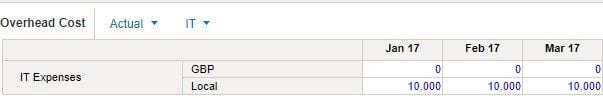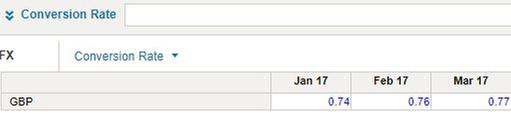# Formula for a specific cellHi,

I have a module with 2 lists: currency (Local and GBP) and list item e.g. Expense. I input data into Local and Expense cell e.g. 1000. I want to calculate GBP amount of that 1000, by multiplying number in Local cell with FX rate.  How do I write formula for the GBP cell?  I find that I can only write formula for the Line Item.Thanks

Andre

Tagged:

•Hi Andre,

I would suggest that you build the following:

1. Module "Conversion rate" + Line item "Value" + List "Currency, Time"

2. Module "Overhead Cost Input" + Line Item "IT Expenses" + List "Time"

Note: Currency is left out, so we're assuming this is an input module referring to Local Currency.

3. Module "Overhead Cost Report" + Line Item "Rate, IT Expenses" + List "Currency, Time"

Note: Rate = if item(Currency) = Currency.Local Currency then 1 else 1/Conversion Rate.Value.

I'm assuming GBP = Local Currency / Conversion Rate

Note: IT Expenses = Rate * Overhead Cost Input.IT Expenses

The above setup allows you to use "Overhead Cost Input" for all your local currency input, while "Overhead Cost Report" allows you to switch between "Local Currency" or any other "Currency".

Thanks,

LipChean